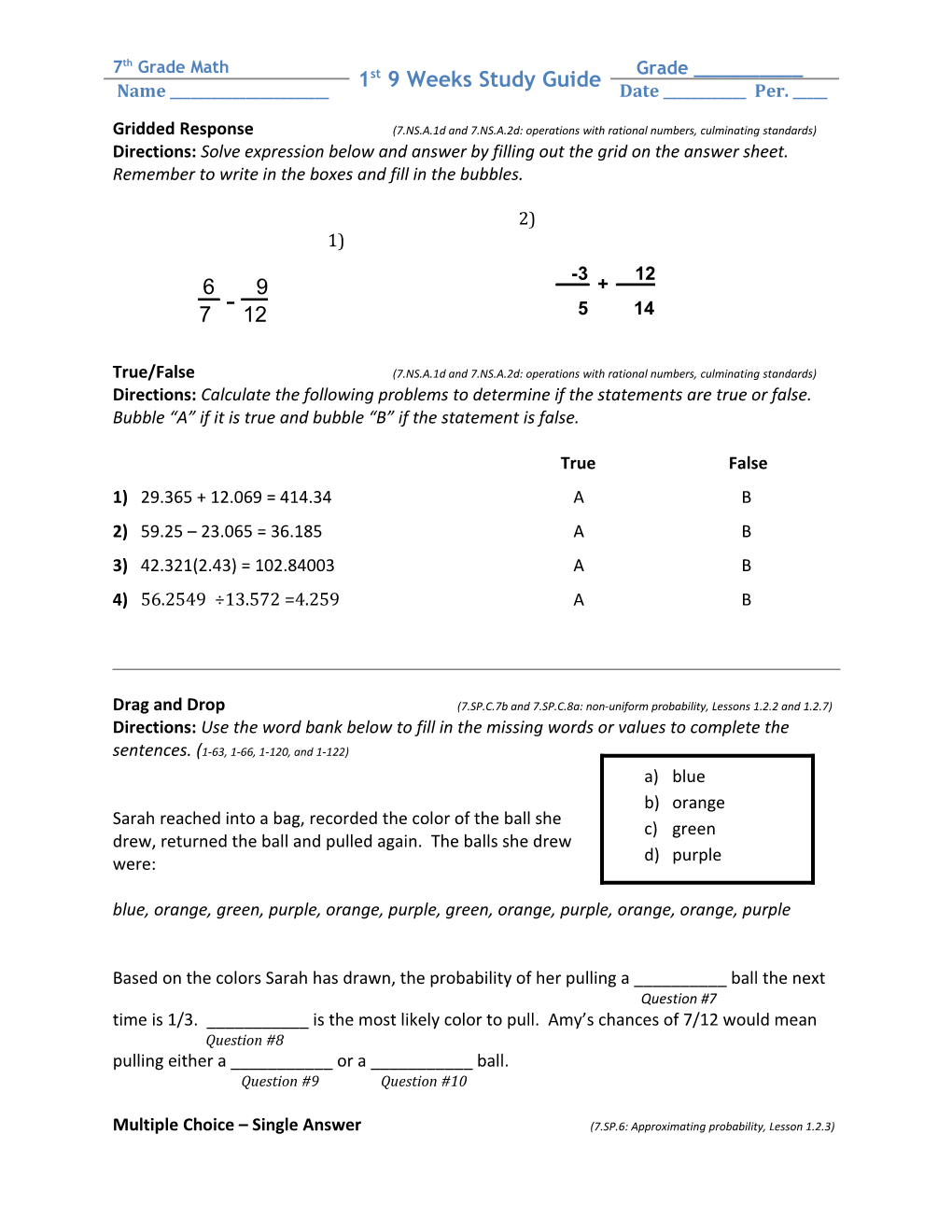Gridded Response (7.NS.A.1D and 7.NS.A.2D: Operations with Rational Numbers, Culminating7th Grade Math / 1st 9 Weeks Study Guide / Grade ______
Name ______ / Date ______Per. _____

Gridded Response (7.NS.A.1d and 7.NS.A.2d: operations with rational numbers, culminating standards)

Directions:Solve expression below and answer by filling out the grid on the answer sheet. Remember to write in the boxes and fill in the bubbles.

-3 / + / 12
5 / 14

2)

1)

6 / - / 9
7 / 12

True/False (7.NS.A.1d and 7.NS.A.2d: operations with rational numbers, culminating standards)

Directions:Calculate the following problems to determine if the statements are true or false. Bubble “A” if it is true and bubble “B” if the statement is false.

TrueFalse

1)29.365 + 12.069 = 414.34 A B

2)59.25 – 23.065 = 36.185 A B

3)42.321(2.43) = 102.84003 A B

4)56.2549 ÷13.572 =4.259 A B

Drag and Drop (7.SP.C.7b and 7.SP.C.8a: non-uniform probability, Lessons 1.2.2 and 1.2.7)

Directions: Use the word bank below to fill in the missing words or values to complete the sentences. (1-63, 1-66, 1-120, and 1-122)

Sarah reached into a bag, recorded the color of the ball she drew, returned the ball and pulled again. The balls she drew were:

blue, orange, green, purple, orange, purple, green, orange, purple, orange, orange, purple

Based on the colors Sarah has drawn, the probability of her pulling a ______ball the next

Question #7

time is 1/3. ______is the most likely color to pull. Amy’s chances of 7/12 would mean

Question #8

pulling either a ______or a ______ball.

Question #9 Question #10

Multiple Choice – Single Answer (7.SP.6: Approximating probability, Lesson 1.2.3)

Directions: Choose the best answer for each of the questions below.

11) There are 2 blue, 2 green and 2 red marbles in the bag. If a blue marble is pulled twelve times out of eighteen, what is the probability that the next marble will be blue?

a) 2/3 c) ½

b) 1/3d) 1

Open-Ended Section(7.SP.6, 7.SP.C.7a, and 7.SP.C.8a: Approximating probability, Lesson 1.2.3)

12) A multiple-choice test consists of questions with 8 possible answers (A, B, C, D, E, F, G, and H). What is the probability of getting a question right by guessing? Express your answer as a fraction, a decimal, and a percent.

______

Drag and Drop(7.NS.1: zero pairs and adding integers, Section 2.2)

Directions: Use the word bank below to fill in the missing words or values to complete the sentences. (1-63, 1-66, 1-120, and 1-122)

Cecil is competing at the Regional Tightrope Competition. His routine is displayed below:

9+6+(−3)+(−3)+(−3)+(-3)+(-4) +(-4)

Based on Cecil’s routine above, his performance ends when he is ______feet

Question #13

on his tightrope. As Cecil was walking his routine, he sneezed and fell off just after his first 4

foot jump, making him fall off the rope at ______feet. Cecil wrote his routine

Question #14

down in a simpler way using distributive property by writing: 9 + 6 + ______(-3) + 2 (______).

Question#15 Question#16

Multiple Choice – Multiple Answers(7.NS.2d: Repeating and terminating, Lessons 1.1.5 and 2.1.2)

Directions: For each problem below, select all that apply.

17) Choose all of the numbers that represent terminating decimals. (1-43)

1
8
A / 12
25
B / 6
5
C / 4
3
D

Multiple Choice – Single Answer(7.NS.1b and 7.NS.2a: Absolute value and distributive property, Section 2.2)

Directions: Choose the best answer for each of the questions below.

18) Evaluate the absolute value of the expression below. (2-45)

- |−8|- |7|

a) 15c) 1

b) -15d) -1

19) Simplify the following expression using the distributive property. ( 2-77)

9(-24 + 62)

a) –342c) -432

b) 342d) 432

Open-Ended Section

20) Use the strategy of your choice to multiply (3 1/5)(4 1/6). (1 point; Lesson 2.2.6)

Drag and Drop(7.NS.1: representing multiplication, Section 3.1)

Directions: Use the word bank below to fill in the missing words or values to complete the sentences. (3-3)

Johnny has arranged some tiles as shown below:

When Adam looked at them he stated very quickly that the first arrangement of tiles could be written as . Marissa said that her second grouping of tiles could be

Question #21

written as . Johnny agreed with both Adam and Marissa and told

Question #22

them that based on his calculations, if he simplified her tiles, the number of tiles left could be represented by the number . Question #23

Multiple Choice – Multiple Answer (7.NS.1 and 2: Integer rules when subtracting or multiplying, Section 3.2)

Directions: For each problem below, select all that apply.

24) Choose all of the choices whose difference will result in a negative value. (3-42 and 3-43)

-13 –10
A / 29 – 49
B /
C / -12.34 – (8.1)
D

25) Choose all of the choices whose product will net a positive value. (Additional Lesson)

-9(-4)
A /
B / (-4.6)2.614(-5.2)
C / -2(-3)(-5)
D

Open-Ended Section

26) Solve the following problems making sure to model your solutions on a number line and with tiles. (4 points; 7.NS.1)

a)-9 – (-7)

b)–4 – 8 + 2 – 5

27) Solve the following problems. (2 points; 7.NS.1 and 7.NS.2)

4( 5– 8 ) + 7 + 2( -2 + 5)²